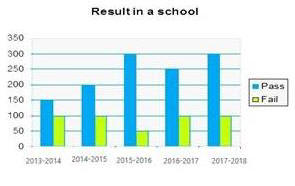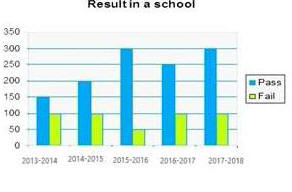# 04 July 2019 – Afternoon-Shift (Quantitative Aptitude)

SSC CHSL (Tier – 1) Online Exam Paper – 2018 “held on 04 JULY 2019”

Afternoon-Shift (Quantitative Aptitude)

Q1. In a ∠ABC, the bisectors of ∠B and ∠C meet at point O within the triangle. If ∠A is given, then which among the given options is true?

Options:

1. ∠BOC=180°- (∠A/2)
2. ∠BOC=90°- (∠A/2)
3. ∠BOC=180°- (∠A)
4. ∠BOC=90°+ (∠A/2)

Q2. If tan x = cot (65°+ 9x), then what is the value of x ?

Options:

1. 2.5°
2. 1.5°
3. 1.0°
4. 2.0°

Q3. 10 years ago, the average age of a family of five members was 38 years. Now, two new members join, whose age difference is 8 years. If the present average age of the family is the same as it was 10 years ago, what is the age (in years) of the new younger member?

Options:

1. 15
2.10
3.9
4.17

Q4. A man bought 2 articles for Rs.2650 each. He sold one article at 10% profit and another at 5% profit. The total profit percentage he earned is :

Options:

1. 7.5%
2. 8.5%
3. 10%
4. 8%

Q5. Let ∠ABC ~ ∠QPR and ar(∠ABC)/ar(∠QPR) = 9/4. If AB = 9cm, BC = 6cm and AC = 12 cm then QR is equal to:

Options:

1. 8cm
2. 16cm
3. 9cm
4. 12cm

Q6. If a 10-digit number 1220x558y2 is divisible by 88, then the value of (x+y) is:

Options:

1. 9
2. 15
3. 7
4. 11

Q7. Rs.60000 invested at a certain rate for a certain even number of years, compounded annually, grows to Rs.63,654. To how much amount would it grow if it is invested at the same rate for the half the period?

Options:

1. Rs.61675
2. Rs.61800
3. Rs.61809
4. Rs.61827

Q8. The simplified value of 5 of 8 – 6 + [(27-3) ÷ 6-4] is:

Options:

1. 114
2. 124
3. 120
4. 116

Q9.  The given Bar graph presents the results in terms of number of students in a school for the five academic years, 2013-2014 to 2017-2018.What is the approximate percentage of students passed during give academic years (correct to the nearest integer) ?

Options:

1. 72%
2. 73%
3. 78%
4. 79%

Q10. The given Bar graph presents the results in terms of number of students in a school for the five academic years, 2013-2014 to 2017-2018.What is the average of failed students in five academic years?

Options:

1. 50
2. 100
3. 75
4. 90

Q11. A, B and C can complete of piece of work in 10,20 and 60 days respectively. Working together, they can  complete the same work in how many days ?

Options:

1. 6
2. 10
3. 5
4. 8

Q12. If  x + X¯4 = 2207, (x>0) then the value of x + x¯ is:

Options:

1. 7
2. 9
3. 19
4. 11

Q13. A number is increased by 30%, then decreased by 25%, and the further increased by 25%. what is the net increase/decrease percent in the number (correct to the nearest integer)

Options:

1. 22% increase
2. 21% decrease
3. 22% decrease
4. 21% increase

Q14. The given Bar graph presents the results in terms of number of students in a school for the five academic years, 2013-2014 to 2017-2018.The difference between the number of students passed and those who failed is the highest in which academic year?

Options:

1. 2015-2016
2. 2016-2017
3. 2017-2018
4. 2014-2015

Q15. If x – 1/x = 10 , then x³- 1/x³ is equal to:

Options:

1. 1000
2. 970
3. 1100
4. 1030

Q16. There was 29% off on bags. A lady bought a bag and got 12% discount for paying in cash. She paid Rs. 312.40. What is the price tag (in Rs) on the bag ?

Options:

1. 625
2. 500
3. 450
4. 600

Q17. Two circle of radii 5 cm and 8 cm intersect at the points A and B. If AB = 8 cm and the distance between the centres of two circles is x cm, then the value of x (to the closet integer) is:

Options:

1. 8
2. 9
3. 11
4. 10

Q18. If cos = 2p / p²+1, then sin is equal to:

Options:

1. 2p / p²-1
2. p²+1/ p²-1
3. p²-1 / p²+1
4. 2p / p²+1

Correct answer : p²-1 / p²+1

Q19. What is the area of a rhombus (in cm²) whose side is 10 cm and the smaller diagonal is 12 cm ?

Options:

1. 50
2. 96
3. 192
4. 120

Q20. If (x – 7)³ + (x – 8)³ + (x + 6)³ = 3(x – 7) (x – 8) (x + 6), then what is the value of x?

Options:

1. 3
2. 8
3. 10
4. 6

Q21. A circle is inscribed in a triangle ABC. It touches sides AB, BC and AC at the points P, Q and R respectively. If BP = 6.5 cm, CQ = 4.5 cm and AR = 5.5 cm, then the perimeter (in cm) of the triangle AABC is:

Options:

1. 16.5
2. 22
3. 33
4. 66

Q22. In triangle ABC, D and E are two points on the sides AB and AC respectively so that DE || BC and AD/BD = 3/4. The ratio of the area of trapezium DECB to the area of ∠ABC is:

Options:

1. 49:33
2. 40:49
3. 33:49
4. 49:40

Q23. A train covers 360 km at a uniform speed. If the speed had been 10 km/h more, it would have taken 3 hours less for the same journey. What is the speed of the train (in km/h)?

Options:

1. 25
2. 40
3. 50
4. 30

Q24. The given Bar graph presents the results in terms of number of students in a school for the five academic years, 2013-2014 to 2017-2018.In which year the percentage increase in the total number of students is the highest in comparison to the previous academic year?

Options:

1. 2016-2017
2. 2014-2015
3. 2015-2014
4. 2017-2018